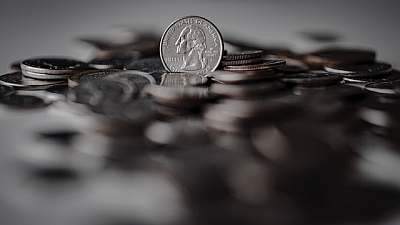# Yield to Maturity Calculator

Instructions: Use this Yield to Maturity Calculator with steps to compute the yield to maturity with this calculator, by indicating the coupon rate paid every period ($$C$$), the bond's current value ($$V$$), the number of periods ($$T$$), and the bond's face value ($$F$$):Coupon $$(C)$$ =Bond Value $$(V)$$ =Number of periods $$(T)$$ =Bond's Face Value $$(F)$$ =

## YTM Calculator

More about the Yield-to-Maturity Calculator so you can better understand how to use this financial calculator.

## What is the Yield-to-Maturity (YTM)?

The $$YTM$$ is the corresponding return that will equate the present value of all cash flows associated to a bond (coupon payments as well as the face value of the bond that is paid at maturity).

Usually, unless we have a zero-coupon bond, the $$YTM$$ cannot computed directly and needs to be solved using Excel, or another scientific calculator.

Excel is a fairly convenient tool for this task, as it has direct and simple tools that make this calculation easy. The problem with it is that the steps are not shown

You can use this YTM calculator to get the calculation shown, with all the relevant steps provided.## Is yield equal to YTM?

This is a question people ask: Is yield equal to YTM? Yield equals YTM only if the bond is held to maturity.

For most other cases, this does not hold up.

## Is a higher YTM better?

Yes, if everything else remains equal, though it ultimately depends on the different parameters of your position, whether you are buying, selling, holding.

There are different parameters that determine the price of a bond, and they are all interlinked by the bond price formula.

### Example: Calculation of the YTM of a firm

Question: Suppose that a bond has a face value of $1000, with a coupon rate of 4% for 10 years, with a current value of$950. Find the yield-to-maturity.

Solution:

So, how do I calculate yield to maturity? This is the information we have been provided with:

 Coupon Paid $$C$$ = $$0.04$$ Bond Value $$V$$ = $$950$$ Number of Periods $$T$$ = $$10$$ Bond's Face Value $$F$$ = $$1000$$

In order to find the Yield to Maturity $$(YTM)$$, we need to find the value $$r$$ so that the following equation is satisfied:

$V = \displaystyle \frac{C}{r}\left( 1 - \frac{1}{(1+r)^T} \right) + \frac{F}{(1+r)^T}$$\Leftrightarrow 950 = \displaystyle \frac{0.04}{r}\left( 1 - \frac{1}{(1+r)^{10}} \right) + \frac{1000}{(1+r)^{10}}$

The above equation cannot be solved directly for $$r$$. Using Excel or another type of calculator we find that $$r = 0.0462$$.

Therefore, given the values for the bond value, coupon paid, number of periods and face value, the Yield to Maturity obtained is $$YTM = 0.0462 = 4.62\%$$.### Price Calculators

You can instead compute the value of a bond using this calculator .

Sometimes, as useful as it is to compute the yield-to-maturity, you may want to assess the price of a stock as well, to assess the difference between difference financial instruments